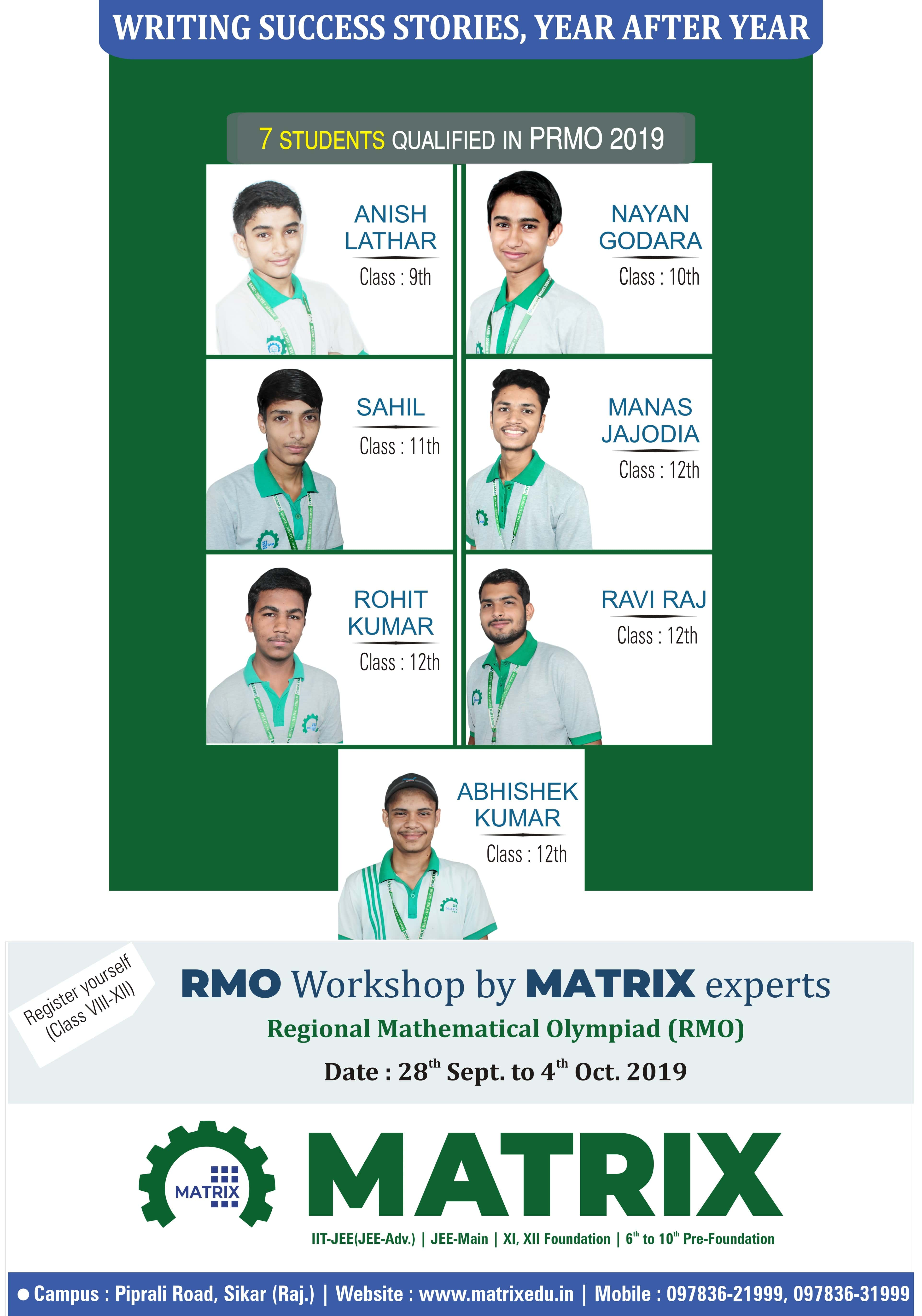# Pre-Regional Mathematical Olympiad (PRMO) Result

The Pre- Regional Mathematical Olympiad is the first level of Mathematics Olympiad. There are six stages of the Maths Olympiad. PRMO is conducted by the Mathematics Teachers’ Association (MTA) while the remaining rounds of the Mathematical Olympiad Program are conducted by Homi Bhabha Centre for Science Education (HBCSE). The exam is conducted for class 8th-12th students.

The Olympiad is conducted in 6 stages which are as follows:

• Stage 1 – Pre-Regional Mathematics Olympiad (PRMO)
• Stage 2 – Regional Mathematical Olympiad (RMO)
• Stage 3 – Indian National Mathematical Olympiad (INMO)
• Stage 4 – International Mathematical Olympiad Training Camp (IMOTC)
• Stage 5 – Pre-Departure Camp
• Stage 6 – International Mathematical Olympiad (IMO)

Our 7 students qualified the exam and 1 student also qualified for Regional Mathematical Olympiad (RMO). Which is highest and first time in Sikar.